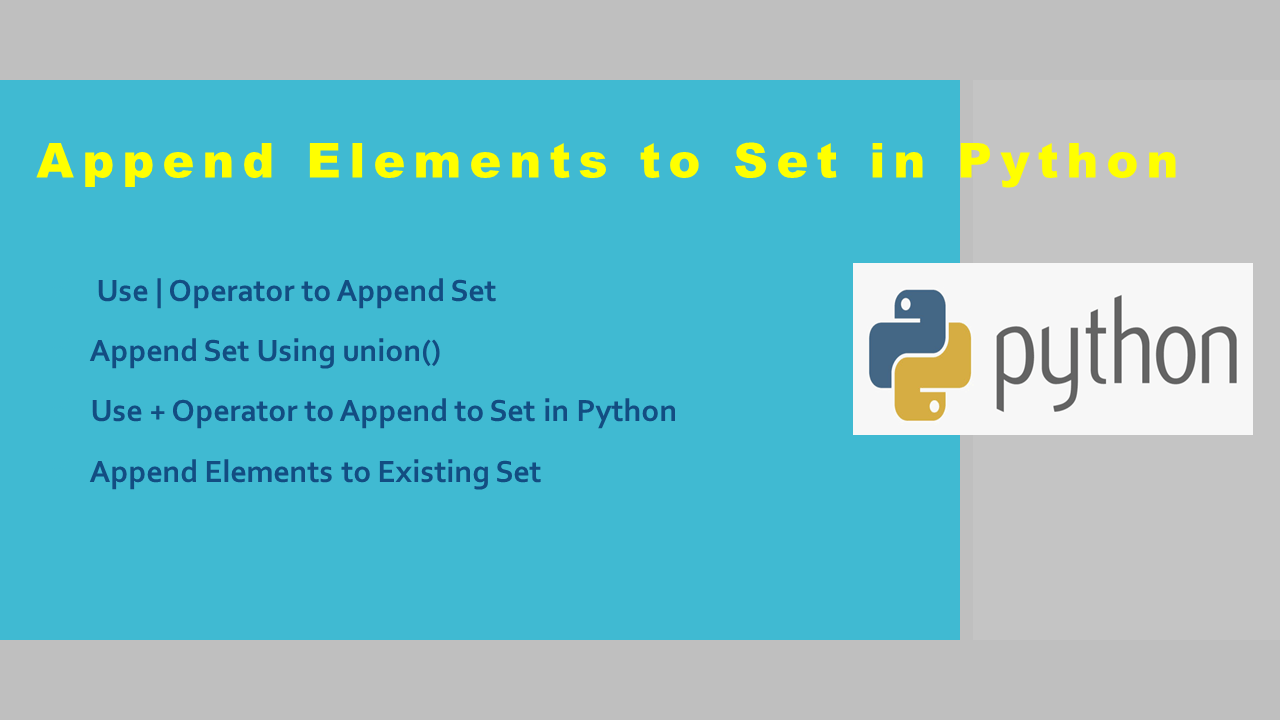# Append Elements to Set in Python

There is no append() method in Python to append elements to the Set. However, there are several ways to append elements to the set. To append elements to the set in Python use the + operator, union() function, and union (|) operator. Besides these, you can also use the add() and update() methods to append elements to the set.

## 1. Quick Examples of Appending Elements to Set

Following are quick examples of appending elements or values to the set.

``````
# Quick Examples

# Append using |
myset = myset1 | myset2 | myset3

# Append using union()
myset = myset1.union(myset2,myset3)

# Append using +
myset = set(list(myset1) + list(myset2))

# Append using update()
myset.update(myset1,myset2)

``````

## 2. Use | Operator to Append Set

The ‘|’ operator refers to the union, which will append the elements to the first set. By using this operator, you can also append multiple sets at a time. Note that this operator returns results without duplicate values.

### 2.1 ‘|’ operator Syntax

``````
# Here, myset is the input set and we can append new sets.
myset | new_set1 | new_set2 | ...
``````

### 2.2 Append Elements to Set using | operator

Let’s use the | operator to append two sets into a single set in Python. This would return a new set that contains all of the elements from `myset1` and `myset2`, without any duplicates.

``````
# Create sets
myset1 = {1,2,3,4,5,6}
myset2 = {7,8,9,1,4}

# Append using operator |
myset = myset1 | myset2
print(myset)

# Output:
# {1, 2, 3, 4, 5, 6, 7, 8, 9}
``````

Let’s also see another example with Strings. Here, I will append elements from three sets into a single set.

``````
# Create sets
set1 = {"one","two","three"}
set2 = {"four","five","six"}
set3 = {"one","four","nine"}

# Append sets with strings using |
myset = set1 | set2 | set3
print(myset)

# Output:
# {'one', 'six', 'two', 'five', 'four', 'nine',
'three'}
``````

## 3. Append Set Using union()

The set.union() is the same as the | operator, which will append the elements to the first set. It can also be possible to append multiple sets at a time.

### 3.1 union() Syntax

Following is the syntax of the union()

``````
# Here, myset is the input set and we can append new sets.
myset.union(new_set1,new_set2,...)
``````

### 3.2 Append Elements to Set using union()

Let’s use the same sets that were created above to append sets.

``````
# Append using union
myset = myset1.union(myset2)
print(myset)

# Output:
# {1, 2, 3, 4, 5, 6, 7, 8, 9}
``````

Similarly, you can append sets with strings. Here, I will append elements from three sets into a single set.

``````
# Append sets with string using union()
myset = set1.union(set2,set3)
print(myset)

# Output:
# {'one', 'six', 'two', 'five', 'four', 'nine', 'three'}
``````

## 4. Use + Operator to Append to Set in Python

The ‘+’ operator is used to append single or multiple sets at a time in Python. We can’t use sets with the + operator hence, we need to convert the set to a list, append lists and convert the result from the list to a set.

### 4.1 ‘+’ operator Syntax

Below is the syntax to use the + operator.

``````
# Here, myset is the input set and we can append new sets.
set(list(myset) + list(new_set1)+ list(new_set2)...)
``````

### 4.2 Examples using + operator

Let’s create two sets with integer values and append them into a single set. To perform the conversion, I will use list() to convert the set to a list and set() to convert the resulting list to a set.

``````
# Append  using +
myset = set(list(myset1) + list(myset2))
print(myset)

# Output:
# {1, 2, 3, 4, 5, 6, 7, 8, 9}
``````

Let’s see another example with strings. Here, I will append elements from three sets into a single set.

``````
# Append  using +
myset = set(list(set1) + list(set2) + list(set3))
print(myset)

# Output:
# {'one', 'six', 'two', 'five', 'four', 'nine', 'three'}
``````

## 5. Append Elements to Existing Set

All the above examples we have seen return a new set after appending values. To append elements to the existing set use set.update() method.

Following is the syntax of the update method.

``````
# Here, myset is the input set.
myset.update(new_set1,new_set2,...)
``````

By using this method, let’s append the elements to the set.

``````
# Append elements to the existing set
myset1.update(myset2)
print(myset1)

set1.update(set2,set3)
print(set1)

# Output:
# {1, 2, 3, 4, 5, 6, 7, 8, 9}
# {'one', 'six', 'two', 'five', 'four', 'nine', 'three'}
``````

## 6. Append Arbitrary Value to Set

By using the set.add() method you can add a single value at a time to the set.

``````